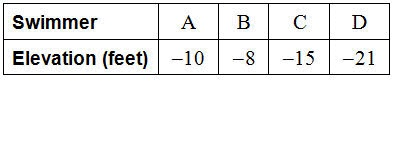Summer Practice - The Number System (Integers)
Complete all questions and submit at end. This is part of your summer math packet. All 5 parts must be completed by September 3, 2019. These questions are to reinforce integers from 6th grade.
Please do not use a calculator.
When answering please submit just a numerical answer. For any answer that requires a negative sign, do not put a space between the sign and the number. (i.e. -4 not - 4)
Last Name *
Your answer
6th grade cluster *
Your answer
First Name *
Your answer
A train is at an elevation of 71 feet, and a submarine is at an elevation of -71 feet. Which of the following is true? *
Which of the following is true given that -4 > -9? *
Which number below best represents a temperature drop of fourteen degrees? *
The average temperature in Hawaii is about 73°F. Which of the following best describes 73°F? *
Which of the following statements is true? *
(-5) + 8 = ? *
Use a number line to help you.
Your answer
8 + (-4) = ? *
Your answer
2 + (-9) = ? *
Your answer
15 + (-3) = ? *
Your answer
(-18) + 7 = ? *
Your answer
(-12) + (-6) = ? *
Your answer
25 + (-15) = ? *
Your answer
(-11) + 5 + (-12) = ? *
Your answer
Numbers that are greater than 0 *
Numbers that are less than 0 *
Tell which number is greater. *
5, -4
Tell which number is greater. *
-7, -16
The table shows the elevation of four swimmers. Which swimmer dives down the farthest? *The temperature at 6 am was -4 degrees. At noon, the temperature was 20 degrees. How many degrees did the temperature change? *
You do not need to put a label, only the value is enough.
Your answer
A scuba diver is 10 feet below the surface and dives down 10 feet more. Represent the divers new elevation as an integer. *
Your answer
How is a temperature of 7 degrees below zero written as an integer? *
Your answer
Never submit passwords through Google Forms.
This form was created inside of Peabody Public Schools. Report Abuse - Terms of Service